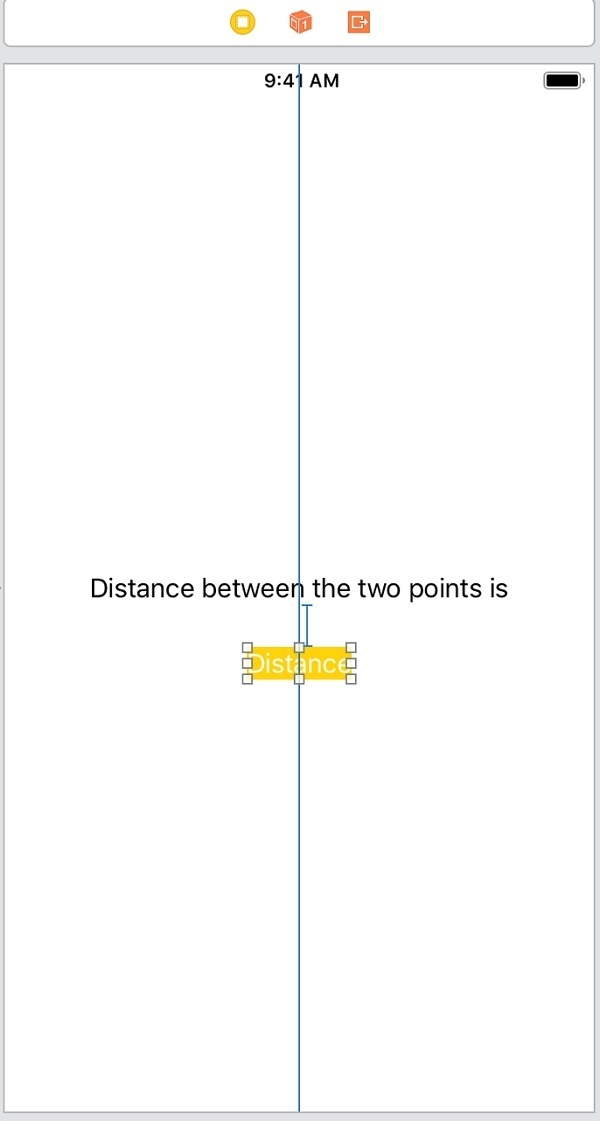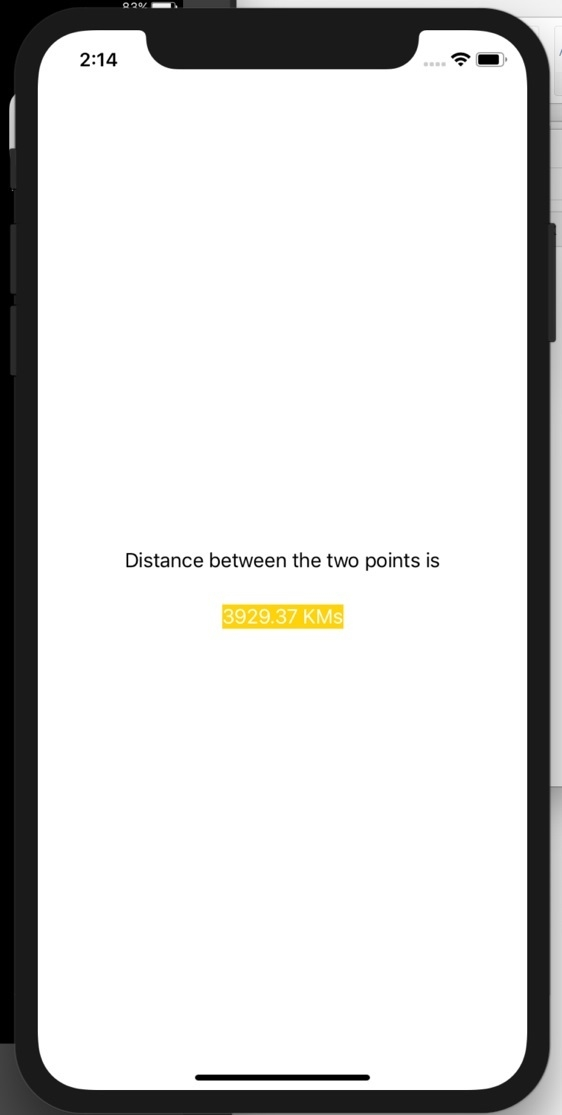# How to get the distance between two geographic locations in iOS using Swift?

In this post we will learn how to calculate the distance between two geo locations.

We will show the distance between two points on a label.

To do so follow the steps below

Step 1 − Open Xcode → New Project → Single View Application → Let’s name it “FindDistance”

Step 2 − Open Main.storyboard and add two labels as shown below.Step 3 − Attach one @IBOutlet for the bottom label. Name it distanceLabel

Step 4 − Import CoreLocation framework in ViewController

Step 5 − Add two points between which we want to find the distance as variables

var firsLocation = CLLocation(latitude:34.54545, longitude:56.64646)
var secondLocation = CLLocation(latitude: 59.326354, longitude: 18.072310)

Step 6 − In viewDidLoad of the view controller add following lines.

let distance = firsLocation.distance(from: secondLocation) / 1000
distanceLabel.text = " \(String(format:"%.02f", distance)) KMs "

Here we are using the ‘distance’ function of the CoreLocation framework. This function returns distance from the point in meters. We are dividing the distance by 1000 to get the distance in Kilo meters.

Step 7 − Run the project you will see the distance on bottom labels. As shown below